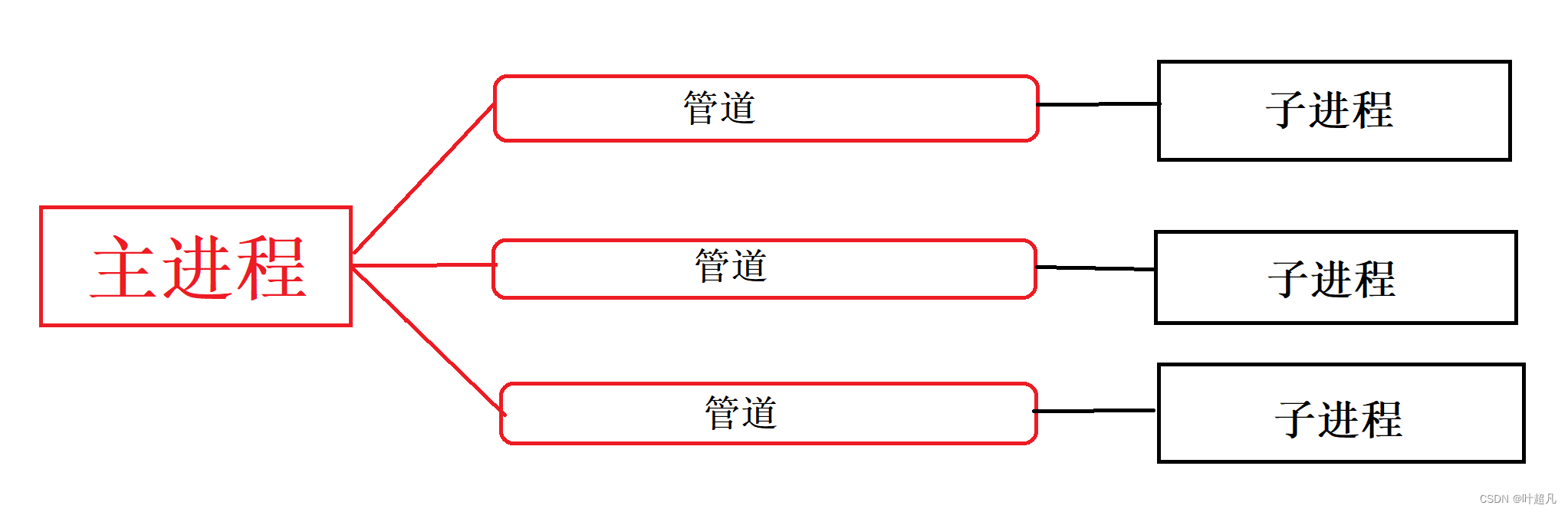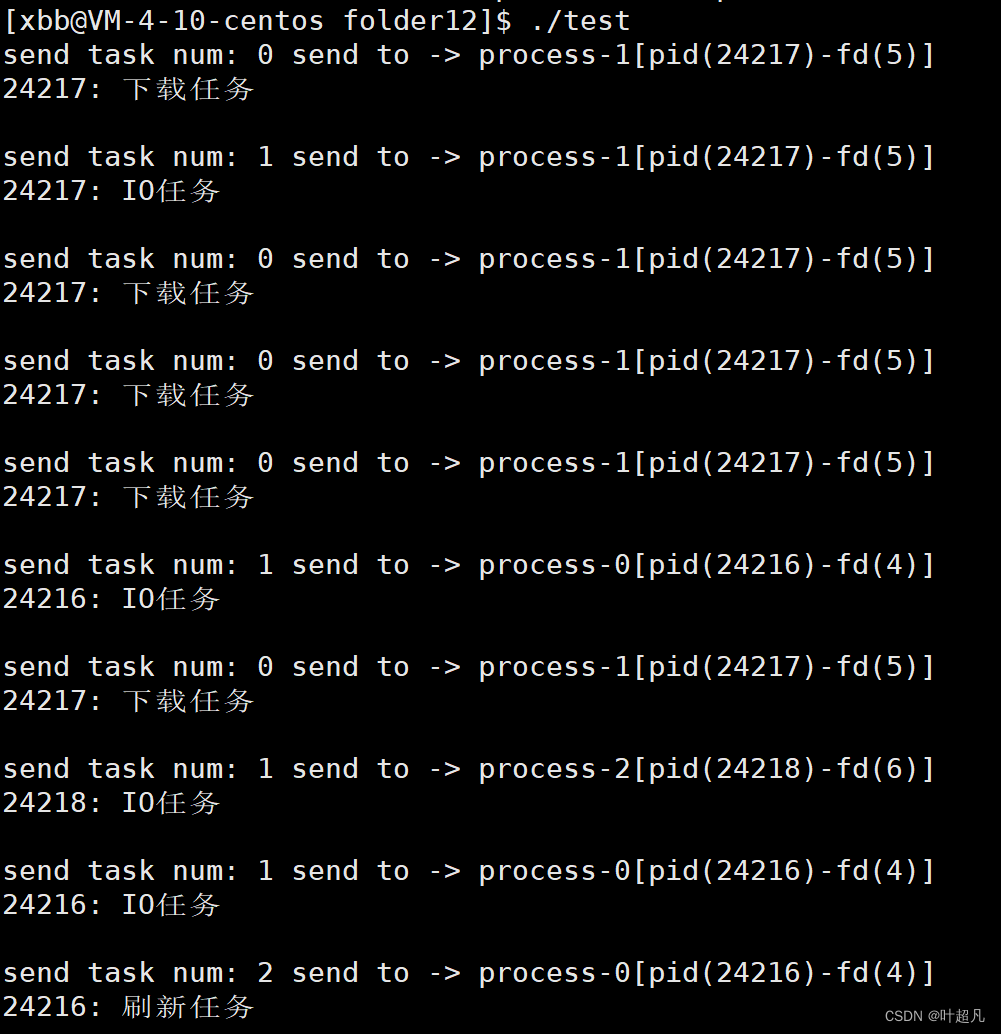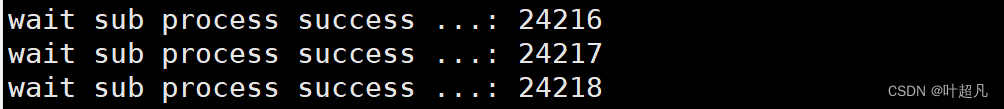# linux入门---用匿名管道实现一个功能

## 前言## 第一步：创建子进程并连接管道

#include<iostream>
#include<unistd.h>
#include<cassert>
#define PROCSS_NUM 3
using namespace std;
int main()
{
for(int i=0;i<PROCSS_NUM;i++)
{
int fds={0};
int n=pipe(fds);
assert(n==0);
(void)n;
pid_t id=fork();
if(id==0)
{
//这里是子进程执行的代码

}
//这里是父进程执行的代码
}
return 0;
}


 if(id==0)
{
//这里是子进程执行的代码
close(fds);
while(true)
{
//读取管道里面的内容

//根据管道的内容执行对应的函数
}
}


class subEp // 描述子进程
{
public:
subEp(pid_t subId, int writeFd)
: subId_(subId), writeFd_(writeFd)
{
char nameBuffer;
snprintf(nameBuffer, sizeof nameBuffer, "process-%d[pid(%d)-fd(%d)]", num++, subId_, writeFd_);
//给子进程创建名字
name_ = nameBuffer;
}

public:
static int num;//用于区别每个子进程
std::string name_;//记录子进程的名字
pid_t subId_;//子进程的id
int writeFd_;//子进程的写端
};


#include<iostream>
#include<unistd.h>
#include<cassert>
#include<string>
#include<vector>
#define PROCSS_NUM 10
using namespace std;
class subEp // 描述子进程
{
public:
subEp(pid_t subId, int writeFd)
: subId_(subId), writeFd_(writeFd)
{
char nameBuffer;
snprintf(nameBuffer, sizeof nameBuffer, "process-%d[pid(%d)-fd(%d)]", num++, subId_, writeFd_);
//给子进程创建名字
name_ = nameBuffer;
}

public:
static int num;//用于区别每个子进程
std::string name_;//记录子进程的名字
pid_t subId_;//子进程的id
int writeFd_;//子进程的写端
};

int subEp::num = 0;
int main()
{
vector<subEP> subs;
for(int i=0;i<PROCSS_NUM;i++)
{
int fds={0};
int n=pipe(fds);
assert(n==0);
(void)n;
pid_t id=fork();
if(id==0)
{
//这里是子进程执行的代码
close(fds);
while(true)
{
//读取管道里面的内容

//根据管道的内容执行对应的函数
}

}
//这里是父进程执行的代码
close[fds];
subs.push_back(subEP(id,fds));

}
return 0;
}


void createSubProcess(std::vector<subEp> *subs, std::vector<func_t> &funcMap)
{
for(int i=0;i<PROCSS_NUM;i++)
{
int fds={0};
int n=pipe(fds);
assert(n==0);
(void)n;
pid_t id=fork();
if(id==0)
{
//这里是子进程执行的代码
close(fds);
while(true)
{
//读取管道里面的内容

//根据管道的内容执行对应的函数
}

}
//这里是父进程执行的代码
close[fds];
subs.push_back(subEP(id,fds));
}
}


## 第二步：建立被执行函数的集合

void downLoadTask()
{
std::cout << getpid() << ": 下载任务\n"
<< std::endl;
sleep(1);
}

{
std::cout << getpid() << ": IO任务\n"
<< std::endl;
sleep(1);
}

{
std::cout << getpid() << ": 刷新任务\n"
<< std::endl;
sleep(1);
}


typedef void (*func_t)();
{
assert(out);
}
int main()
{
vector<func_t> funcMap;
vector<subEP> subs;
//创建子进程
createSubProcess(subs,funcmap);
return 0;
}


## 第三步：父进程发送信息

srand((unsigned long)time(nullptr) ^ getpid() ^ 0x171237 ^ rand() % 1234);


void loadBlanceContrl(const std::vector<subEp> &subs, const std::vector<func_t> &funcMap, int count)
{

}


void loadBlanceContrl(const std::vector<subEp> &subs, const std::vector<func_t> &funcMap, int count)
{
int processnum=subs.num();
bool forver =(count==0? true:false);
while(true)
{

}
}


void sendTask(const subEp &process, int taskNum)
{

}
void loadBlanceContrl(const std::vector<subEp> &subs, const std::vector<func_t> &funcMap, int count)
{
int processnum=subs.num();
bool forever =(count==0? true:false);
while(true)
{
int subIdx=rand()%processnum;
}
}


void sendTask(const subEp &process, int taskNum)
{
cout << "send task num: " << taskNum << " send to -> " << process.name_ << endl;
assert(n==sizeof(int));
(void)n;
}


void loadBlanceContrl(const std::vector<subEp> &subs, const std::vector<func_t> &funcMap, int count)
{
int processnum=subs.num();
bool forever =(count==0? true:false);
while(true)
{
int subIdx=rand()%processnum;
}
if(!forever)
{
--count;
if(count==0)
{
break;
}
}
for(int i=0;i<process;i++)
{
close(subs[i].writeFd_);
}
}


## 第四步：信号的接收和实行函数

void createSubProcess(std::vector<subEp> *subs, std::vector<func_t> &funcMap)
{
for(int i=0;i<PROCSS_NUM;i++)
{
int fds={0};
int n=pipe(fds);
assert(n==0);
(void)n;
pid_t id=fork();
if(id==0)
{
//这里是子进程执行的代码
close(fds);
while(true)
{
//读取管道里面的内容

//根据管道的内容执行对应的函数
}

}
//这里是父进程执行的代码
close[fds];
subs.push_back(subEP(id,fds));
}
}


int recvTask(int readFd)
{

}


int recvTask(int readFd)
{
int code=0;
if(s==4)    {return code;}
else if(s<=0)   {return -1;}
else    {return 0}
}


 while(true)
{
//读取管道里面的内容
//根据管道的内容执行对应的函数
if(commondCode>=0&&commondCode<funcMap.size())
{
funcMap[commandCode]();
}
else if(commandCode == -1)
{
break;
}
}


## 第五步：子进程等待函数

int main()
{
vector<func_t> funcMap;
vector<subEP> subs;
//创建子进程
createSubProcess(subs,funcmap);
return 0;
}


int main()
{
vector<func_t> funcMap;
vector<subEP> subs;
//创建子进程
createSubProcess(subs,funcmap);
int count=10;
return 0;
}


void waitProcess(std::vector<subEp> processes)
{
int processnum = processes.size();
for(int i = 0; i < processnum; i++)
{
waitpid(processes[i].subId_, nullptr, 0);
std::cout << "wait sub process success ...: " << processes[i].subId_ << std::endl;
}
}
int main()
{
vector<func_t> funcMap;
vector<subEP> subs;
//创建子进程
createSubProcess(subs,funcmap);
int count=10;
waitProcess(subs);
return 0;
}


## 程序的测试

PROCSS_NUM的值为3，并且count的值为10，所以当前程序运行起来之后会创建出3个子进程然后一共执行10个任务，那么运行的现象就是这样：## 程序的bug

void createSubProcess(std::vector<subEp> *subs, std::vector<func_t> &funcMap)
{
std::vector<int> deleteFd;
for(int i=0;i<PROCSS_NUM;i++)
{
int fds={0};
int n=pipe(fds);
assert(n==0);
(void)n;
pid_t id=fork();
if(id==0)
{
cout<<"创建了子进程"<< endl;
for(int i = 0; i < deleteFd.size(); i++) close(deleteFd[i]);
//这里是子进程执行的代码
close(fds);
while(true)
{
//读取管道里面的内容
//根据管道的内容执行对应的函数
if(commondCode>=0&&commondCode<funcMap.size())
{
funcMap[commondCode]();
}
else if(commondCode == -1)
{
break;
}
}
exit(0);
}
//这里是父进程执行的代码
close(fds);
subEp sub(id,fds);
subs->push_back(sub);
deleteFd.push_back(fds);
}
}


#include <iostream>
#include <string>
#include <vector>
#include <cstdlib>
#include <cassert>
#include <ctime>
#include <sys/types.h>
#include <sys/wait.h>
#include <unistd.h>
#define PROCSS_NUM 3
using namespace std;
typedef void (*func_t)();
class subEp // 描述子进程
{
public:
subEp(pid_t subId, int writeFd)
: subId_(subId), writeFd_(writeFd)
{
char nameBuffer;
snprintf(nameBuffer, sizeof nameBuffer, "process-%d[pid(%d)-fd(%d)]", num++, subId_, writeFd_);
//给子进程创建名字
name_ = nameBuffer;
}
public:
static int num;//用于区别每个子进程
std::string name_;//记录子进程的名字
pid_t subId_;//子进程的id
int writeFd_;//子进程的写端
};
int subEp ::num =0;
{
std::cout << getpid() << ": 下载任务\n"
<< std::endl;
sleep(1);
}

{
std::cout << getpid() << ": IO任务\n"
<< std::endl;
sleep(1);
}

{
std::cout << getpid() << ": 刷新任务\n"
<< std::endl;
sleep(1);
}
{
int code=0;
if(s==4)    {return code;}
else if(s<=0)   {return -1;}
else    {return 0;}
}
void createSubProcess(std::vector<subEp> *subs, std::vector<func_t> &funcMap)
{
std::vector<int> deleteFd;
for(int i=0;i<PROCSS_NUM;i++)
{
int fds={0};
int n=pipe(fds);
assert(n==0);
(void)n;
pid_t id=fork();
if(id==0)
{
cout<<"创建了子进程"<< endl;
for(int i = 0; i < deleteFd.size(); i++) close(deleteFd[i]);
//这里是子进程执行的代码
close(fds);
while(true)
{
//读取管道里面的内容
//根据管道的内容执行对应的函数
if(commondCode>=0&&commondCode<funcMap.size())
{
funcMap[commondCode]();
}
else if(commondCode == -1)
{
break;
}
}
exit(0);
}
//这里是父进程执行的代码
close(fds);
subEp sub(id,fds);
subs->push_back(sub);
deleteFd.push_back(fds);
}
}

{
assert(out);
}
{
cout << "send task num: " << taskNum << " send to -> " << process.name_ << endl;
assert(n==sizeof(int));
(void)n;
}
void loadBlanceContrl(const std::vector<subEp> &subs, const std::vector<func_t> &funcMap, int count)
{
int processnum=subs.size();
bool forever =(count==0? true:false);
while(true)
{
int subIdx=rand()%processnum;
sleep(1);
if(!forever)
{
--count;
if(count==0)
{
break;
}
}
}
for(int i=0;i<processnum;i++)
{
close(subs[i].writeFd_);
}
}
void waitProcess(std::vector<subEp> processes)
{
int processnum = processes.size();
for(int i = 0; i < processnum; i++)
{
waitpid(processes[i].subId_, nullptr, 0);
std::cout << "wait sub process success ...: " << processes[i].subId_ << std::endl;
}
}
int main()
{
srand((unsigned long)time(nullptr) ^ getpid() ^ 0x171237 ^ rand() % 1234);
vector<func_t> funcMap;
vector<subEp> subs;
//创建子进程
createSubProcess(&subs,funcMap);
int count=10;